Signal Processing (scipy.signal)¶

The signal processing toolbox currently contains some filtering functions, a limited set of filter design tools, and a few B-spline interpolation algorithms for one- and two-dimensional data. While the B-spline algorithms could technically be placed under the interpolation category, they are included here because they only work with equally-spaced data and make heavy use of filter-theory and transfer-function formalism to provide a fast B-spline transform. To understand this section you will need to understand that a signal in SciPy is an array of real or complex numbers.

B-splines¶

A B-spline is an approximation of a continuous function over a finite- domain in terms of B-spline coefficients and knot points. If the knot- points are equally spaced with spacing $$\Delta x$$ , then the B-spline approximation to a 1-dimensional function is the finite-basis expansion.

$y\left(x\right)\approx\sum_{j}c_{j}\beta^{o}\left(\frac{x}{\Delta x}-j\right).$

In two dimensions with knot-spacing $$\Delta x$$ and $$\Delta y$$ , the function representation is

$z\left(x,y\right)\approx\sum_{j}\sum_{k}c_{jk}\beta^{o}\left(\frac{x}{\Delta x}-j\right)\beta^{o}\left(\frac{y}{\Delta y}-k\right).$

In these expressions, $$\beta^{o}\left(\cdot\right)$$ is the space-limited B-spline basis function of order, $$o$$ . The requirement of equally-spaced knot-points and equally-spaced data points, allows the development of fast (inverse-filtering) algorithms for determining the coefficients, $$c_{j}$$ , from sample-values, $$y_{n}$$ . Unlike the general spline interpolation algorithms, these algorithms can quickly find the spline coefficients for large images.

The advantage of representing a set of samples via B-spline basis functions is that continuous-domain operators (derivatives, re- sampling, integral, etc.) which assume that the data samples are drawn from an underlying continuous function can be computed with relative ease from the spline coefficients. For example, the second-derivative of a spline is

$y{}^{\prime\prime}\left(x\right)=\frac{1}{\Delta x^{2}}\sum_{j}c_{j}\beta^{o\prime\prime}\left(\frac{x}{\Delta x}-j\right).$

Using the property of B-splines that

$\frac{d^{2}\beta^{o}\left(w\right)}{dw^{2}}=\beta^{o-2}\left(w+1\right)-2\beta^{o-2}\left(w\right)+\beta^{o-2}\left(w-1\right)$

it can be seen that

$y^{\prime\prime}\left(x\right)=\frac{1}{\Delta x^{2}}\sum_{j}c_{j}\left[\beta^{o-2}\left(\frac{x}{\Delta x}-j+1\right)-2\beta^{o-2}\left(\frac{x}{\Delta x}-j\right)+\beta^{o-2}\left(\frac{x}{\Delta x}-j-1\right)\right].$

If $$o=3$$ , then at the sample points,

\begin{eqnarray*} \Delta x^{2}\left.y^{\prime}\left(x\right)\right|_{x=n\Delta x} & = & \sum_{j}c_{j}\delta_{n-j+1}-2c_{j}\delta_{n-j}+c_{j}\delta_{n-j-1},\\ & = & c_{n+1}-2c_{n}+c_{n-1}.\end{eqnarray*}

Thus, the second-derivative signal can be easily calculated from the spline fit. if desired, smoothing splines can be found to make the second-derivative less sensitive to random-errors.

The savvy reader will have already noticed that the data samples are related to the knot coefficients via a convolution operator, so that simple convolution with the sampled B-spline function recovers the original data from the spline coefficients. The output of convolutions can change depending on how boundaries are handled (this becomes increasingly more important as the number of dimensions in the data- set increases). The algorithms relating to B-splines in the signal- processing sub package assume mirror-symmetric boundary conditions. Thus, spline coefficients are computed based on that assumption, and data-samples can be recovered exactly from the spline coefficients by assuming them to be mirror-symmetric also.

Currently the package provides functions for determining second- and third- order cubic spline coefficients from equally spaced samples in one- and two- dimensions (qspline1d, qspline2d, cspline1d, cspline2d). The package also supplies a function ( bspline ) for evaluating the bspline basis function, $$\beta^{o}\left(x\right)$$ for arbitrary order and $$x.$$ For large $$o$$ , the B-spline basis function can be approximated well by a zero-mean Gaussian function with standard-deviation equal to $$\sigma_{o}=\left(o+1\right)/12$$ :

$\beta^{o}\left(x\right)\approx\frac{1}{\sqrt{2\pi\sigma_{o}^{2}}}\exp\left(-\frac{x^{2}}{2\sigma_{o}}\right).$

A function to compute this Gaussian for arbitrary $$x$$ and $$o$$ is also available ( gauss_spline ). The following code and Figure uses spline-filtering to compute an edge-image (the second-derivative of a smoothed spline) of a raccoon’s face which is an array returned by the command misc.face. The command sepfir2d was used to apply a separable two-dimensional FIR filter with mirror- symmetric boundary conditions to the spline coefficients. This function is ideally suited for reconstructing samples from spline coefficients and is faster than convolve2d which convolves arbitrary two-dimensional filters and allows for choosing mirror-symmetric boundary conditions.

>>> import numpy as np
>>> from scipy import signal, misc
>>> import matplotlib.pyplot as plt
>>> image = misc.face(gray=True).astype(np.float32)
>>> derfilt = np.array([1.0, -2, 1.0], dtype=np.float32)
>>> ck = signal.cspline2d(image, 8.0)
>>> deriv = (signal.sepfir2d(ck, derfilt, ) +
...          signal.sepfir2d(ck, , derfilt))

Alternatively we could have done:

laplacian = np.array([[0,1,0], [1,-4,1], [0,1,0]], dtype=np.float32)
deriv2 = signal.convolve2d(ck,laplacian,mode='same',boundary='symm')
>>> plt.figure()
>>> plt.imshow(image)
>>> plt.gray()
>>> plt.title('Original image')
>>> plt.show()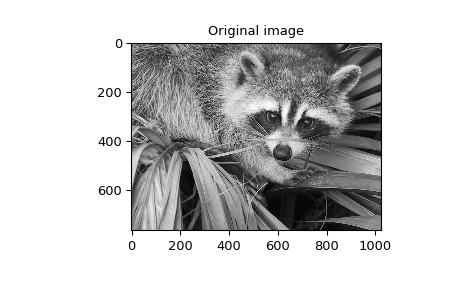>>> plt.figure()
>>> plt.imshow(deriv)
>>> plt.gray()
>>> plt.title('Output of spline edge filter')
>>> plt.show()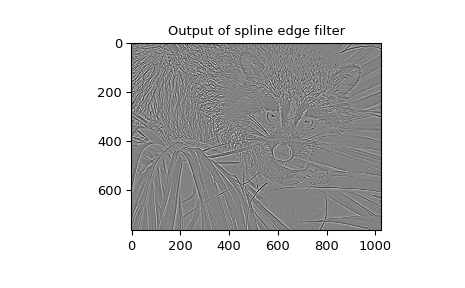Filtering¶

Filtering is a generic name for any system that modifies an input signal in some way. In SciPy a signal can be thought of as a Numpy array. There are different kinds of filters for different kinds of operations. There are two broad kinds of filtering operations: linear and non-linear. Linear filters can always be reduced to multiplication of the flattened Numpy array by an appropriate matrix resulting in another flattened Numpy array. Of course, this is not usually the best way to compute the filter as the matrices and vectors involved may be huge. For example filtering a $$512 \times 512$$ image with this method would require multiplication of a $$512^2 \times 512^2$$ matrix with a $$512^2$$ vector. Just trying to store the $$512^2 \times 512^2$$ matrix using a standard Numpy array would require $$68,719,476,736$$ elements. At 4 bytes per element this would require $$256\textrm{GB}$$ of memory. In most applications most of the elements of this matrix are zero and a different method for computing the output of the filter is employed.

Convolution/Correlation¶

Many linear filters also have the property of shift-invariance. This means that the filtering operation is the same at different locations in the signal and it implies that the filtering matrix can be constructed from knowledge of one row (or column) of the matrix alone. In this case, the matrix multiplication can be accomplished using Fourier transforms.

Let $$x\left[n\right]$$ define a one-dimensional signal indexed by the integer $$n.$$ Full convolution of two one-dimensional signals can be expressed as

$y\left[n\right]=\sum_{k=-\infty}^{\infty}x\left[k\right]h\left[n-k\right].$

This equation can only be implemented directly if we limit the sequences to finite support sequences that can be stored in a computer, choose $$n=0$$ to be the starting point of both sequences, let $$K+1$$ be that value for which $$x\left[n\right]=0$$ for all $$n\geq K+1$$ and $$M+1$$ be that value for which $$h\left[n\right]=0$$ for all $$n\geq M+1$$ , then the discrete convolution expression is

$y\left[n\right]=\sum_{k=\max\left(n-M,0\right)}^{\min\left(n,K\right)}x\left[k\right]h\left[n-k\right].$

For convenience assume $$K\geq M.$$ Then, more explicitly the output of this operation is

\begin{eqnarray*} y\left[0\right] & = & x\left[0\right]h\left[0\right]\\ y\left[1\right] & = & x\left[0\right]h\left[1\right]+x\left[1\right]h\left[0\right]\\ y\left[2\right] & = & x\left[0\right]h\left[2\right]+x\left[1\right]h\left[1\right]+x\left[2\right]h\left[0\right]\\ \vdots & \vdots & \vdots\\ y\left[M\right] & = & x\left[0\right]h\left[M\right]+x\left[1\right]h\left[M-1\right]+\cdots+x\left[M\right]h\left[0\right]\\ y\left[M+1\right] & = & x\left[1\right]h\left[M\right]+x\left[2\right]h\left[M-1\right]+\cdots+x\left[M+1\right]h\left[0\right]\\ \vdots & \vdots & \vdots\\ y\left[K\right] & = & x\left[K-M\right]h\left[M\right]+\cdots+x\left[K\right]h\left[0\right]\\ y\left[K+1\right] & = & x\left[K+1-M\right]h\left[M\right]+\cdots+x\left[K\right]h\left[1\right]\\ \vdots & \vdots & \vdots\\ y\left[K+M-1\right] & = & x\left[K-1\right]h\left[M\right]+x\left[K\right]h\left[M-1\right]\\ y\left[K+M\right] & = & x\left[K\right]h\left[M\right].\end{eqnarray*}

Thus, the full discrete convolution of two finite sequences of lengths $$K+1$$ and $$M+1$$ respectively results in a finite sequence of length $$K+M+1=\left(K+1\right)+\left(M+1\right)-1.$$

One dimensional convolution is implemented in SciPy with the function convolve. This function takes as inputs the signals $$x,$$ $$h$$ , and two optional flags ‘mode’ and ‘method’ and returns the signal $$y.$$

The first optional flag ‘mode’ allows for specification of which part of the output signal to return. The default value of ‘full’ returns the entire signal. If the flag has a value of ‘same’ then only the middle $$K$$ values are returned starting at $$y\left[\left\lfloor \frac{M-1}{2}\right\rfloor \right]$$ so that the output has the same length as the first input. If the flag has a value of ‘valid’ then only the middle $$K-M+1=\left(K+1\right)-\left(M+1\right)+1$$ output values are returned where $$z$$ depends on all of the values of the smallest input from $$h\left[0\right]$$ to $$h\left[M\right].$$ In other words only the values $$y\left[M\right]$$ to $$y\left[K\right]$$ inclusive are returned.

The second optional flag ‘method’ determines how the convolution is computed, either through the Fourier transform approach with fftconvolve or through the direct method. By default, it selects the expected faster method. The Fourier transform method has order $$O(N\log N)$$ while the direct method has order $$O(N^2)$$. Depending on the big O constant and the value of $$N$$, one of these two methods may be faster. The default value ‘auto’ performs a rough calculation and chooses the expected faster method, while the values ‘direct’ and ‘fft’ force computation with the other two methods.

The code below shows a simple example for convolution of 2 sequences

>>> x = np.array([1.0, 2.0, 3.0])
>>> h = np.array([0.0, 1.0, 0.0, 0.0, 0.0])
>>> signal.convolve(x, h)
array([ 0.,  1.,  2.,  3.,  0.,  0.,  0.])
>>> signal.convolve(x, h, 'same')
array([ 2.,  3.,  0.])

This same function convolve can actually take $$N$$ -dimensional arrays as inputs and will return the $$N$$ -dimensional convolution of the two arrays as is shown in the code example below. The same input flags are available for that case as well.

>>> x = np.array([[1., 1., 0., 0.], [1., 1., 0., 0.], [0., 0., 0., 0.], [0., 0., 0., 0.]])
>>> h = np.array([[1., 0., 0., 0.], [0., 0., 0., 0.], [0., 0., 1., 0.], [0., 0., 0., 0.]])
>>> signal.convolve(x, h)
array([[ 1.,  1.,  0.,  0.,  0.,  0.,  0.],
[ 1.,  1.,  0.,  0.,  0.,  0.,  0.],
[ 0.,  0.,  1.,  1.,  0.,  0.,  0.],
[ 0.,  0.,  1.,  1.,  0.,  0.,  0.],
[ 0.,  0.,  0.,  0.,  0.,  0.,  0.],
[ 0.,  0.,  0.,  0.,  0.,  0.,  0.],
[ 0.,  0.,  0.,  0.,  0.,  0.,  0.]])

Correlation is very similar to convolution except for the minus sign becomes a plus sign. Thus

$w\left[n\right]=\sum_{k=-\infty}^{\infty}y\left[k\right]x\left[n+k\right]$

is the (cross) correlation of the signals $$y$$ and $$x.$$ For finite-length signals with $$y\left[n\right]=0$$ outside of the range $$\left[0,K\right]$$ and $$x\left[n\right]=0$$ outside of the range $$\left[0,M\right],$$ the summation can simplify to

$w\left[n\right]=\sum_{k=\max\left(0,-n\right)}^{\min\left(K,M-n\right)}y\left[k\right]x\left[n+k\right].$

Assuming again that $$K\geq M$$ this is

\begin{eqnarray*} w\left[-K\right] & = & y\left[K\right]x\left[0\right]\\ w\left[-K+1\right] & = & y\left[K-1\right]x\left[0\right]+y\left[K\right]x\left[1\right]\\ \vdots & \vdots & \vdots\\ w\left[M-K\right] & = & y\left[K-M\right]x\left[0\right]+y\left[K-M+1\right]x\left[1\right]+\cdots+y\left[K\right]x\left[M\right]\\ w\left[M-K+1\right] & = & y\left[K-M-1\right]x\left[0\right]+\cdots+y\left[K-1\right]x\left[M\right]\\ \vdots & \vdots & \vdots\\ w\left[-1\right] & = & y\left[1\right]x\left[0\right]+y\left[2\right]x\left[1\right]+\cdots+y\left[M+1\right]x\left[M\right]\\ w\left[0\right] & = & y\left[0\right]x\left[0\right]+y\left[1\right]x\left[1\right]+\cdots+y\left[M\right]x\left[M\right]\\ w\left[1\right] & = & y\left[0\right]x\left[1\right]+y\left[1\right]x\left[2\right]+\cdots+y\left[M-1\right]x\left[M\right]\\ w\left[2\right] & = & y\left[0\right]x\left[2\right]+y\left[1\right]x\left[3\right]+\cdots+y\left[M-2\right]x\left[M\right]\\ \vdots & \vdots & \vdots\\ w\left[M-1\right] & = & y\left[0\right]x\left[M-1\right]+y\left[1\right]x\left[M\right]\\ w\left[M\right] & = & y\left[0\right]x\left[M\right].\end{eqnarray*}

The SciPy function correlate implements this operation. Equivalent flags are available for this operation to return the full $$K+M+1$$ length sequence (‘full’) or a sequence with the same size as the largest sequence starting at $$w\left[-K+\left\lfloor \frac{M-1}{2}\right\rfloor \right]$$ (‘same’) or a sequence where the values depend on all the values of the smallest sequence (‘valid’). This final option returns the $$K-M+1$$ values $$w\left[M-K\right]$$ to $$w\left[0\right]$$ inclusive.

The function correlate can also take arbitrary $$N$$ -dimensional arrays as input and return the $$N$$ -dimensional convolution of the two arrays on output.

When $$N=2,$$ correlate and/or convolve can be used to construct arbitrary image filters to perform actions such as blurring, enhancing, and edge-detection for an image.

>>> import numpy as np
>>> from scipy import signal, misc
>>> import matplotlib.pyplot as plt
>>> image = misc.face(gray=True)
>>> w = np.zeros((50, 50))
>>> w = 1.0
>>> w = 1.0
>>> image_new = signal.fftconvolve(image, w)
>>> plt.figure()
>>> plt.imshow(image)
>>> plt.gray()
>>> plt.title('Original image')
>>> plt.show()>>> plt.figure()
>>> plt.imshow(image_new)
>>> plt.gray()
>>> plt.title('Filtered image')
>>> plt.show()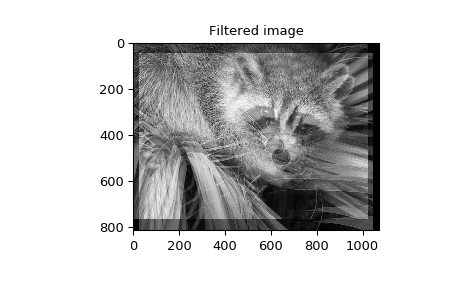Calculating the convolution in the time domain as above is mainly used for filtering when one of the signals is much smaller than the other ( $$K\gg M$$ ), otherwise linear filtering is more efficiently calculated in the frequency domain provided by the function fftconvolve. By default, convolve estimates the fastest method using choose_conv_method.

If the filter function $$w[n,m]$$ can be factored according to

$h[n, m] = h_1[n] h_2[m],$

convolution can be calculated by means of the function sepfir2d. As an example we consider a Gaussian filter gaussian

$h[n, m] \propto e^{-x^2-y^2} = e^{-x^2} e^{-y^2}$

which is often used for blurring.

>>> import numpy as np
>>> from scipy import signal, misc
>>> import matplotlib.pyplot as plt
>>> image = misc.ascent()
>>> w = signal.gaussian(50, 10.0)
>>> image_new = signal.sepfir2d(image, w, w)
>>> plt.figure()
>>> plt.imshow(image)
>>> plt.gray()
>>> plt.title('Original image')
>>> plt.show()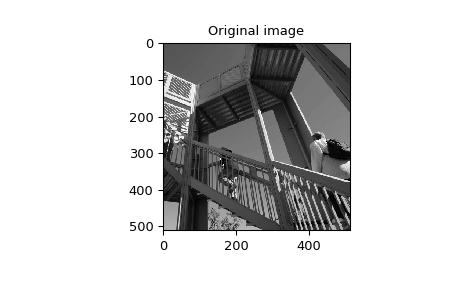>>> plt.figure()
>>> plt.imshow(image_new)
>>> plt.gray()
>>> plt.title('Filtered image')
>>> plt.show()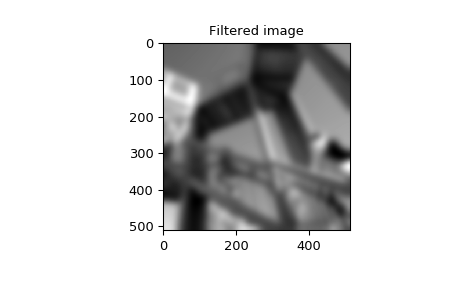Difference-equation filtering¶

A general class of linear one-dimensional filters (that includes convolution filters) are filters described by the difference equation

$\sum_{k=0}^{N}a_{k}y\left[n-k\right]=\sum_{k=0}^{M}b_{k}x\left[n-k\right]$

where $$x\left[n\right]$$ is the input sequence and $$y\left[n\right]$$ is the output sequence. If we assume initial rest so that $$y\left[n\right]=0$$ for $$n<0$$ , then this kind of filter can be implemented using convolution. However, the convolution filter sequence $$h\left[n\right]$$ could be infinite if $$a_{k}\neq0$$ for $$k\geq1.$$ In addition, this general class of linear filter allows initial conditions to be placed on $$y\left[n\right]$$ for $$n<0$$ resulting in a filter that cannot be expressed using convolution.

The difference equation filter can be thought of as finding $$y\left[n\right]$$ recursively in terms of it’s previous values

$a_{0}y\left[n\right]=-a_{1}y\left[n-1\right]-\cdots-a_{N}y\left[n-N\right]+\cdots+b_{0}x\left[n\right]+\cdots+b_{M}x\left[n-M\right].$

Often $$a_{0}=1$$ is chosen for normalization. The implementation in SciPy of this general difference equation filter is a little more complicated then would be implied by the previous equation. It is implemented so that only one signal needs to be delayed. The actual implementation equations are (assuming $$a_{0}=1$$ ).

\begin{eqnarray*} y\left[n\right] & = & b_{0}x\left[n\right]+z_{0}\left[n-1\right]\\ z_{0}\left[n\right] & = & b_{1}x\left[n\right]+z_{1}\left[n-1\right]-a_{1}y\left[n\right]\\ z_{1}\left[n\right] & = & b_{2}x\left[n\right]+z_{2}\left[n-1\right]-a_{2}y\left[n\right]\\ \vdots & \vdots & \vdots\\ z_{K-2}\left[n\right] & = & b_{K-1}x\left[n\right]+z_{K-1}\left[n-1\right]-a_{K-1}y\left[n\right]\\ z_{K-1}\left[n\right] & = & b_{K}x\left[n\right]-a_{K}y\left[n\right],\end{eqnarray*}

where $$K=\max\left(N,M\right).$$ Note that $$b_{K}=0$$ if $$K>M$$ and $$a_{K}=0$$ if $$K>N.$$ In this way, the output at time $$n$$ depends only on the input at time $$n$$ and the value of $$z_{0}$$ at the previous time. This can always be calculated as long as the $$K$$ values $$z_{0}\left[n-1\right]\ldots z_{K-1}\left[n-1\right]$$ are computed and stored at each time step.

The difference-equation filter is called using the command lfilter in SciPy. This command takes as inputs the vector $$b,$$ the vector, $$a,$$ a signal $$x$$ and returns the vector $$y$$ (the same length as $$x$$ ) computed using the equation given above. If $$x$$ is $$N$$ -dimensional, then the filter is computed along the axis provided. If, desired, initial conditions providing the values of $$z_{0}\left[-1\right]$$ to $$z_{K-1}\left[-1\right]$$ can be provided or else it will be assumed that they are all zero. If initial conditions are provided, then the final conditions on the intermediate variables are also returned. These could be used, for example, to restart the calculation in the same state.

Sometimes it is more convenient to express the initial conditions in terms of the signals $$x\left[n\right]$$ and $$y\left[n\right].$$ In other words, perhaps you have the values of $$x\left[-M\right]$$ to $$x\left[-1\right]$$ and the values of $$y\left[-N\right]$$ to $$y\left[-1\right]$$ and would like to determine what values of $$z_{m}\left[-1\right]$$ should be delivered as initial conditions to the difference-equation filter. It is not difficult to show that for $$0\leq m<K,$$

$z_{m}\left[n\right]=\sum_{p=0}^{K-m-1}\left(b_{m+p+1}x\left[n-p\right]-a_{m+p+1}y\left[n-p\right]\right).$

Using this formula we can find the initial condition vector $$z_{0}\left[-1\right]$$ to $$z_{K-1}\left[-1\right]$$ given initial conditions on $$y$$ (and $$x$$ ). The command lfiltic performs this function.

As an example consider the following system:

$y[n] = \frac{1}{2} x[n] + \frac{1}{4} x[n-1] + \frac{1}{3} y[n-1]$

The code calculates the signal $$y[n]$$ for a given signal $$x[n]$$; first for initial conditions $$y[-1] = 0$$ (default case), then for $$y[-1] = 2$$ by means of lfiltic.

>>> import numpy as np
>>> from scipy import signal
>>> x = np.array([1., 0., 0., 0.])
>>> b = np.array([1.0/2, 1.0/4])
>>> a = np.array([1.0, -1.0/3])
>>> signal.lfilter(b, a, x)
array([0.5, 0.41666667, 0.13888889, 0.0462963])
>>> zi = signal.lfiltic(b, a, y=[2.])
>>> signal.lfilter(b, a, x, zi=zi)
(array([ 1.16666667,  0.63888889,  0.21296296,  0.07098765]), array([0.02366]))

Note that the output signal $$y[n]$$ has the same length as the length as the input signal $$x[n]$$.

Analysis of Linear Systems¶

Linear system described a linear difference equation can be fully described by the coefficient vectors a and b as was done above; an alternative representation is to provide a factor $$k$$, $$N_z$$ zeros $$z_k$$ and $$N_p$$ poles $$p_k$$, respectively, to describe the system by means of its transfer function $$H(z)$$ according to

$H(z) = k \frac{ (z-z_1)(z-z_2)...(z-z_{N_z})}{ (z-p_1)(z-p_2)...(z-p_{N_p})}$

This alternative representation can be obtain with the scipy function tf2zpk; the inverse is provided by zpk2tf.

For the example from above we have

>>> b = np.array([1.0/2, 1.0/4])
>>> a = np.array([1.0, -1.0/3])
>>> signal.tf2zpk(b, a)
(array([-0.5]), array([ 0.33333333]), 0.5)

i.e. the system has a zero at $$z=-1/2$$ and a pole at $$z=1/3$$.

The scipy function freqz allows calculation of the frequency response of a system described by the coefficients $$a_k$$ and $$b_k$$. See the help of the freqz function of a comprehensive example.

Filter Design¶

Time-discrete filters can be classified into finite response (FIR) filters and infinite response (IIR) filters. FIR filters can provide a linear phase response, whereas IIR filters cannot. Scipy provides functions for designing both types of filters.

FIR Filter¶

The function firwin designs filters according to the window method. Depending on the provided arguments, the function returns different filter types (e.g. low-pass, band-pass…).

The example below designs a low-pass and a band-stop filter, respectively.

>>> import numpy as np
>>> import scipy.signal as signal
>>> import matplotlib.pyplot as plt
>>> b1 = signal.firwin(40, 0.5)
>>> b2 = signal.firwin(41, [0.3, 0.8])
>>> w1, h1 = signal.freqz(b1)
>>> w2, h2 = signal.freqz(b2)
>>> plt.title('Digital filter frequency response')
>>> plt.plot(w1, 20*np.log10(np.abs(h1)), 'b')
>>> plt.plot(w2, 20*np.log10(np.abs(h2)), 'r')
>>> plt.ylabel('Amplitude Response (dB)')
>>> plt.grid()
>>> plt.show()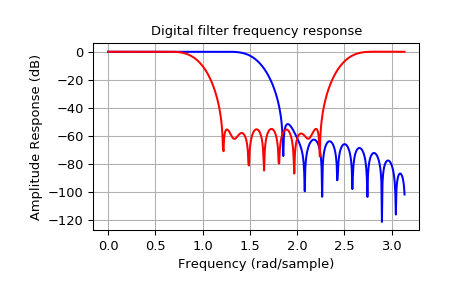Note that firwin uses per default a normalized frequency defined such that the value $$1$$ corresponds to the Nyquist frequency, whereas the function freqz is defined such that the value $$\pi$$ corresponds to the Nyquist frequency.

The function firwin2 allows design of almost arbitrary frequency responses by specifying an array of corner frequencies and corresponding gains, respectively.

The example below designs a filter with such an arbitrary amplitude response.

>>> import numpy as np
>>> import scipy.signal as signal
>>> import matplotlib.pyplot as plt
>>> b = signal.firwin2(150, [0.0, 0.3, 0.6, 1.0], [1.0, 2.0, 0.5, 0.0])
>>> w, h = signal.freqz(b)
>>> plt.title('Digital filter frequency response')
>>> plt.plot(w, np.abs(h))
>>> plt.title('Digital filter frequency response')
>>> plt.ylabel('Amplitude Response')
>>> plt.grid()
>>> plt.show()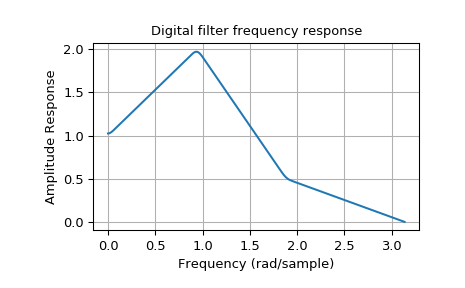Note the linear scaling of the y-axis and the different definition of the Nyquist frequency in firwin2 and freqz (as explained above).

IIR Filter¶

Scipy provides two functions to directly design IIR iirdesign and iirfilter where the filter type (e.g. elliptic) is passed as an argument and several more filter design functions for specific filter types; e.g. ellip.

The example below designs an elliptic low-pass filter with defined passband and stopband ripple, respectively. Note the much lower filter order (order 4) compared with the FIR filters from the examples above in order to reach the same stop-band attenuation of $$\approx 60$$ dB.

>>> import numpy as np
>>> import scipy.signal as signal
>>> import matplotlib.pyplot as plt
>>> b, a = signal.iirfilter(4, Wn=0.2, rp=5, rs=60, btype='lowpass', ftype='ellip')
>>> w, h = signal.freqz(b, a)
>>> plt.title('Digital filter frequency response')
>>> plt.plot(w, 20*np.log10(np.abs(h)))
>>> plt.title('Digital filter frequency response')
>>> plt.ylabel('Amplitude Response [dB]')
>>> plt.grid()
>>> plt.show()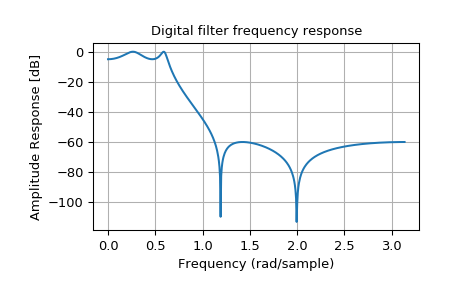Filter Coefficients¶

Filter coefficients can be stored in several different formats:

• ‘ba’ or ‘tf’ = transfer function coefficients
• ‘zpk’ = zeros, poles, and overall gain
• ‘ss’ = state-space system representation
• ‘sos’ = transfer function coefficients of second-order sections

Functions such as tf2zpk and zpk2ss can convert between them.

Transfer function representation¶

The ba or tf format is a 2-tuple (b, a) representing a transfer function, where b is a length M+1 array of coefficients of the M-order numerator polynomial, and a is a length N+1 array of coefficients of the N-order denominator, as positive, descending powers of the transfer function variable. So the tuple of $$b = [b_0, b_1, ..., b_M]$$ and $$a =[a_0, a_1, ..., a_N]$$ can represent an analog filter of the form:

$H(s) = \frac {b_0 s^M + b_1 s^{(M-1)} + \cdots + b_M} {a_0 s^N + a_1 s^{(N-1)} + \cdots + a_N} = \frac {\sum_{i=0}^M b_i s^{(M-i)}} {\sum_{i=0}^N a_i s^{(N-i)}}$

or a discrete-time filter of the form:

$H(z) = \frac {b_0 z^M + b_1 z^{(M-1)} + \cdots + b_M} {a_0 z^N + a_1 z^{(N-1)} + \cdots + a_N} = \frac {\sum_{i=0}^M b_i z^{(M-i)}} {\sum_{i=0}^N a_i z^{(N-i)}}$

This “positive powers” form is found more commonly in controls engineering. If M and N are equal (which is true for all filters generated by the bilinear transform), then this happens to be equivalent to the “negative powers” discrete-time form preferred in DSP:

$H(z) = \frac {b_0 + b_1 z^{-1} + \cdots + b_M z^{-M}} {a_0 + a_1 z^{-1} + \cdots + a_N z^{-N}} = \frac {\sum_{i=0}^M b_i z^{-i}} {\sum_{i=0}^N a_i z^{-i}}$

Although this is true for common filters, remember that this is not true in the general case. If M and N are not equal, the discrete-time transfer function coefficients must first be converted to the “positive powers” form before finding the poles and zeros.

This representation suffers from numerical error at higher orders, so other formats are preferred when possible.

Zeros and poles representation¶

The zpk format is a 3-tuple (z, p, k), where z is an M-length array of the complex zeros of the transfer function $$z = [z_0, z_1, ..., z_{M-1}]$$, p is an N-length array of the complex poles of the transfer function $$p = [p_0, p_1, ..., p_{N-1}]$$, and k is a scalar gain. These represent the digital transfer function:

$H(z) = k \cdot \frac {(z - z_0) (z - z_1) \cdots (z - z_{(M-1)})} {(z - p_0) (z - p_1) \cdots (z - p_{(N-1)})} = k \frac {\prod_{i=0}^{M-1} (z - z_i)} {\prod_{i=0}^{N-1} (z - p_i)}$

or the analog transfer function:

$H(s) = k \cdot \frac {(s - z_0) (s - z_1) \cdots (s - z_{(M-1)})} {(s - p_0) (s - p_1) \cdots (s - p_{(N-1)})} = k \frac {\prod_{i=0}^{M-1} (s - z_i)} {\prod_{i=0}^{N-1} (s - p_i)}$

Although the sets of roots are stored as ordered NumPy arrays, their ordering does not matter; ([-1, -2], [-3, -4], 1) is the same filter as ([-2, -1], [-4, -3], 1).

State-space system representation¶

The ss format is a 4-tuple of arrays (A, B, C, D) representing the state-space of an N-order digital/discrete-time system of the form:

$\begin{split}\mathbf{x}[k+1] = A \mathbf{x}[k] + B \mathbf{u}[k]\\ \mathbf{y}[k] = C \mathbf{x}[k] + D \mathbf{u}[k]\end{split}$

or a continuous/analog system of the form:

$\begin{split}\dot{\mathbf{x}}(t) = A \mathbf{x}(t) + B \mathbf{u}(t)\\ \mathbf{y}(t) = C \mathbf{x}(t) + D \mathbf{u}(t)\end{split}$

with P inputs, Q outputs and N state variables, where:

• x is the state vector
• y is the output vector of length Q
• u is the input vector of length P
• A is the state matrix, with shape (N, N)
• B is the input matrix with shape (N, P)
• C is the output matrix with shape (Q, N)
• D is the feedthrough or feedforward matrix with shape (Q, P). (In cases where the system does not have a direct feedthrough, all values in D are zero.)

State-space is the most general representation, and the only one that allows for multiple-input, multiple-output (MIMO) systems. There are multiple state-space representations for a given transfer function. Specifically, the “controllable canonical form” and “observable canonical form” have the same coefficients as the tf representation, and therefore suffer from the same numerical errors.

Second-order sections representation¶

The sos format is a single 2D array of shape (n_sections, 6), representing a sequence of second-order transfer functions which, when cascaded in series, realize a higher-order filter with minimal numerical error. Each row corresponds to a second-order tf representation, with the first three columns providing the numerator coefficients and the last three providing the denominator coefficients:

$[b_0, b_1, b_2, a_0, a_1, a_2]$

The coefficients are typically normalized such that $$a_0$$ is always 1. The section order is usually not important with floating-point computation; the filter output will be the same regardless.

Filter transformations¶

The IIR filter design functions first generate a prototype analog lowpass filter with a normalized cutoff frequency of 1 rad/sec. This is then transformed into other frequencies and band types using the following substitutions:

Type Transformation
lp2lp $$s \rightarrow \frac{s}{\omega_0}$$
lp2hp $$s \rightarrow \frac{\omega_0}{s}$$
lp2bp $$s \rightarrow \frac{s^2 + {\omega_0}^2}{s \cdot \mathrm{BW}}$$
lp2bs $$s \rightarrow \frac{s \cdot \mathrm{BW}}{s^2 + {\omega_0}^2}$$

Here, $$\omega_0$$ is the new cutoff or center frequency, and $$\mathrm{BW}$$ is the bandwidth. These preserve symmetry on a logarithmic frequency axis.

To convert the transformed analog filter into a digital filter, the bilinear transform is used, which makes the following substitution:

$s \rightarrow \frac{2}{T} \frac{z - 1}{z + 1}$

where T is the sampling time (the inverse of the sampling frequency).

Other filters¶

The signal processing package provides many more filters as well.

Median Filter¶

A median filter is commonly applied when noise is markedly non-Gaussian or when it is desired to preserve edges. The median filter works by sorting all of the array pixel values in a rectangular region surrounding the point of interest. The sample median of this list of neighborhood pixel values is used as the value for the output array. The sample median is the middle array value in a sorted list of neighborhood values. If there are an even number of elements in the neighborhood, then the average of the middle two values is used as the median. A general purpose median filter that works on N-dimensional arrays is medfilt . A specialized version that works only for two-dimensional arrays is available as medfilt2d .

Order Filter¶

A median filter is a specific example of a more general class of filters called order filters. To compute the output at a particular pixel, all order filters use the array values in a region surrounding that pixel. These array values are sorted and then one of them is selected as the output value. For the median filter, the sample median of the list of array values is used as the output. A general order filter allows the user to select which of the sorted values will be used as the output. So, for example one could choose to pick the maximum in the list or the minimum. The order filter takes an additional argument besides the input array and the region mask that specifies which of the elements in the sorted list of neighbor array values should be used as the output. The command to perform an order filter is order_filter.

Wiener filter¶

The Wiener filter is a simple deblurring filter for denoising images. This is not the Wiener filter commonly described in image reconstruction problems but instead it is a simple, local-mean filter. Let $$x$$ be the input signal, then the output is

$\begin{split}y=\left\{ \begin{array}{cc} \frac{\sigma^{2}}{\sigma_{x}^{2}}m_{x}+\left(1-\frac{\sigma^{2}}{\sigma_{x}^{2}}\right)x & \sigma_{x}^{2}\geq\sigma^{2},\\ m_{x} & \sigma_{x}^{2}<\sigma^{2},\end{array}\right.\end{split}$

where $$m_{x}$$ is the local estimate of the mean and $$\sigma_{x}^{2}$$ is the local estimate of the variance. The window for these estimates is an optional input parameter (default is $$3\times3$$ ). The parameter $$\sigma^{2}$$ is a threshold noise parameter. If $$\sigma$$ is not given then it is estimated as the average of the local variances.

Hilbert filter¶

The Hilbert transform constructs the complex-valued analytic signal from a real signal. For example if $$x=\cos\omega n$$ then $$y=\textrm{hilbert}\left(x\right)$$ would return (except near the edges) $$y=\exp\left(j\omega n\right).$$ In the frequency domain, the hilbert transform performs

$Y=X\cdot H$

where $$H$$ is 2 for positive frequencies, $$0$$ for negative frequencies and $$1$$ for zero-frequencies.

Analog Filter Design¶

The functions iirdesign, iirfilter, and the filter design functions for specific filter types (e.g. ellip) all have a flag analog which allows design of analog filters as well.

The example below designs an analog (IIR) filter, obtains via tf2zpk the poles and zeros and plots them in the complex s-plane. The zeros at $$\omega \approx 150$$ and $$\omega \approx 300$$ can be clearly seen in the amplitude response.

>>> import numpy as np
>>> import scipy.signal as signal
>>> import matplotlib.pyplot as plt
>>> b, a = signal.iirdesign(wp=100, ws=200, gpass=2.0, gstop=40., analog=True)
>>> w, h = signal.freqs(b, a)
>>> plt.title('Analog filter frequency response')
>>> plt.plot(w, 20*np.log10(np.abs(h)))
>>> plt.ylabel('Amplitude Response [dB]')
>>> plt.xlabel('Frequency')
>>> plt.grid()
>>> plt.show()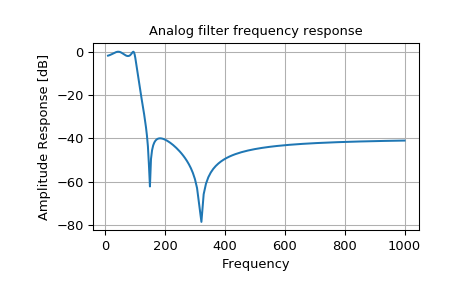>>> z, p, k = signal.tf2zpk(b, a)
>>> plt.plot(np.real(z), np.imag(z), 'xb')
>>> plt.plot(np.real(p), np.imag(p), 'or')
>>> plt.legend(['Zeros', 'Poles'], loc=2)
>>> plt.title('Pole / Zero Plot')
>>> plt.ylabel('Real')
>>> plt.xlabel('Imaginary')
>>> plt.grid()
>>> plt.show()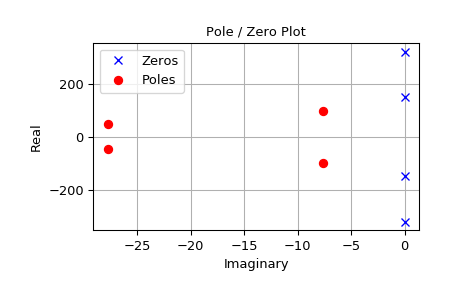Spectral Analysis¶

Periodogram Measurements¶

The scipy function periodogram provides a method to estimate the spectral density using the periodogram method.

The example below calculates the periodogram of a sine signal in white Gaussian noise.

>>> import numpy as np
>>> import scipy.signal as signal
>>> import matplotlib.pyplot as plt
>>> fs = 10e3
>>> N = 1e5
>>> amp = 2*np.sqrt(2)
>>> freq = 1270.0
>>> noise_power = 0.001 * fs / 2
>>> time = np.arange(N) / fs
>>> x = amp*np.sin(2*np.pi*freq*time)
>>> x += np.random.normal(scale=np.sqrt(noise_power), size=time.shape)
>>> f, Pper_spec = signal.periodogram(x, fs, 'flattop', scaling='spectrum')
>>> plt.semilogy(f, Pper_spec)
>>> plt.xlabel('frequency [Hz]')
>>> plt.ylabel('PSD')
>>> plt.grid()
>>> plt.show()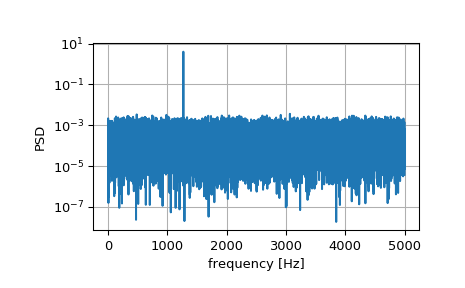Spectral Analysis using Welch’s Method¶

An improved method, especially with respect to noise immunity, is Welch’s method which is implemented by the scipy function welch.

The example below estimates the spectrum using Welch’s method and uses the same parameters as the example above. Note the much smoother noise floor of the spectrogram.

>>> import numpy as np
>>> import scipy.signal as signal
>>> import matplotlib.pyplot as plt
>>> fs = 10e3
>>> N = 1e5
>>> amp = 2*np.sqrt(2)
>>> freq = 1270.0
>>> noise_power = 0.001 * fs / 2
>>> time = np.arange(N) / fs
>>> x = amp*np.sin(2*np.pi*freq*time)
>>> x += np.random.normal(scale=np.sqrt(noise_power), size=time.shape)
>>> f, Pwelch_spec = signal.welch(x, fs, scaling='spectrum')
>>> plt.semilogy(f, Pwelch_spec)
>>> plt.xlabel('frequency [Hz]')
>>> plt.ylabel('PSD')
>>> plt.grid()
>>> plt.show()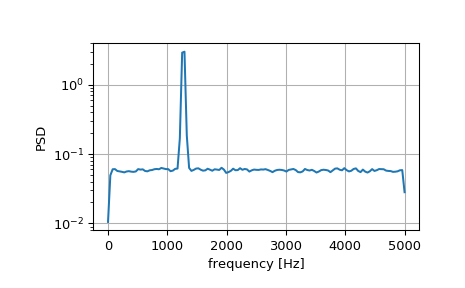Lomb-Scargle Periodograms (lombscargle)¶

Least-squares spectral analysis (LSSA) is a method of estimating a frequency spectrum, based on a least squares fit of sinusoids to data samples, similar to Fourier analysis. Fourier analysis, the most used spectral method in science, generally boosts long-periodic noise in long gapped records; LSSA mitigates such problems.

The Lomb-Scargle method performs spectral analysis on unevenly sampled data and is known to be a powerful way to find, and test the significance of, weak periodic signals.

For a time series comprising $$N_{t}$$ measurements $$X_{j}\equiv X(t_{j})$$ sampled at times $$t_{j}$$ where $$(j = 1, \ldots, N_{t})$$, assumed to have been scaled and shifted such that its mean is zero and its variance is unity, the normalized Lomb-Scargle periodogram at frequency $$f$$ is

$P_{n}(f) \frac{1}{2}\left\{\frac{\left[\sum_{j}^{N_{t}}X_{j}\cos\omega(t_{j}-\tau)\right]^{2}}{\sum_{j}^{N_{t}}\cos^{2}\omega(t_{j}-\tau)}+\frac{\left[\sum_{j}^{N_{t}}X_{j}\sin\omega(t_{j}-\tau)\right]^{2}}{\sum_{j}^{N_{t}}\sin^{2}\omega(t_{j}-\tau)}\right\}.$

Here, $$\omega \equiv 2\pi f$$ is the angular frequency. The frequency dependent time offset $$\tau$$ is given by

$\tan 2\omega\tau = \frac{\sum_{j}^{N_{t}}\sin 2\omega t_{j}}{\sum_{j}^{N_{t}}\cos 2\omega t_{j}}.$

The lombscargle function calculates the periodogram using a slightly modified algorithm due to Townsend  which allows the periodogram to be calculated using only a single pass through the input arrays for each frequency.

The equation is refactored as:

$P_{n}(f) = \frac{1}{2}\left[\frac{(c_{\tau}XC + s_{\tau}XS)^{2}}{c_{\tau}^{2}CC + 2c_{\tau}s_{\tau}CS + s_{\tau}^{2}SS} + \frac{(c_{\tau}XS - s_{\tau}XC)^{2}}{c_{\tau}^{2}SS - 2c_{\tau}s_{\tau}CS + s_{\tau}^{2}CC}\right]$

and

$\tan 2\omega\tau = \frac{2CS}{CC-SS}.$

Here,

$c_{\tau} = \cos\omega\tau,\qquad s_{\tau} = \sin\omega\tau$

while the sums are

$\begin{split}XC &= \sum_{j}^{N_{t}} X_{j}\cos\omega t_{j}\\ XS &= \sum_{j}^{N_{t}} X_{j}\sin\omega t_{j}\\ CC &= \sum_{j}^{N_{t}} \cos^{2}\omega t_{j}\\ SS &= \sum_{j}^{N_{t}} \sin^{2}\omega t_{j}\\ CS &= \sum_{j}^{N_{t}} \cos\omega t_{j}\sin\omega t_{j}.\end{split}$

This requires $$N_{f}(2N_{t}+3)$$ trigonometric function evaluations giving a factor of $$\sim 2$$ speed increase over the straightforward implementation.

Detrend¶

Scipy provides the function detrend to remove a constant or linear trend in a data series in order to see effect of higher order.

The example below removes the constant and linear trend of a 2-nd order polynomial time series and plots the remaining signal components.

>>> import numpy as np
>>> import scipy.signal as signal
>>> import matplotlib.pyplot as plt
>>> t = np.linspace(-10, 10, 20)
>>> y = 1 + t + 0.01*t**2
>>> yconst = signal.detrend(y, type='constant')
>>> ylin = signal.detrend(y, type='linear')
>>> plt.plot(t, y, '-rx')
>>> plt.plot(t, yconst, '-bo')
>>> plt.plot(t, ylin, '-k+')
>>> plt.grid()
>>> plt.legend(['signal', 'const. detrend', 'linear detrend'])
>>> plt.show()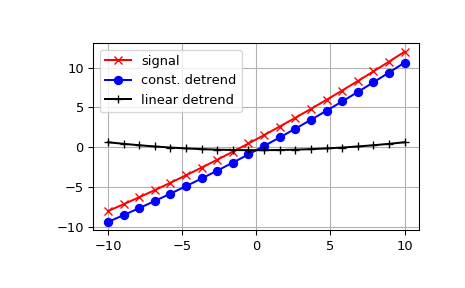References

Some further reading and related software:

  N.R. Lomb “Least-squares frequency analysis of unequally spaced data”, Astrophysics and Space Science, vol 39, pp. 447-462, 1976
  J.D. Scargle “Studies in astronomical time series analysis. II - Statistical aspects of spectral analysis of unevenly spaced data”, The Astrophysical Journal, vol 263, pp. 835-853, 1982
  R.H.D. Townsend, “Fast calculation of the Lomb-Scargle periodogram using graphics processing units.”, The Astrophysical Journal Supplement Series, vol 191, pp. 247-253, 2010